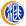# 四版币开创多个“历史第一”500)this.width=500 align=center hspace=10 vspace=10 rel=nofollow/>

500)this.width=500 align=center hspace=10 vspace=10 rel=nofollow/使用党和国家领导人人像作为钞票正面主景，既反映了广大人民群众对老一代革命家的热爱和敬仰，又反映了党和国家领导人对历史的尊重。同时四位伟人为中国和全世界人民熟悉，具有着较高的防伪性能。500)this.width=500 align=center hspace=10 vspace=10 rel=nofollow/>

500)this.width=500 align=center hspace=10 vspace=10 rel=nofollow/500)this.width=500 align=center hspace=10 vspace=10 rel=nofollow/>

500)this.width=500 align=center hspace=10 vspace=10 rel=nofollow/在票面1元以上的主币上增印上了盲文面额数字，以便盲人识别。体现了党和国家对残疾人的重视、尊重与关怀。500)this.width=500 align=center hspace=10 vspace=10 rel=nofollow/>

500)this.width=500 align=center hspace=10 vspace=10 rel=nofollow/第一次行长之章更换了颜色。第四套人民币的印章均印在背面，制版工艺又有了改进，将印章做在印钞图案印版上，印章颜色随票面主色，如票面主色为绿色，印章的颜色也就是绿色。500)this.width=500 align=center hspace=10 vspace=10 rel=nofollow/>

500)this.width=500 align=center hspace=10 vspace=10 rel=nofollow/第一次出现固定人像水印。第四套人民币100元券、50元券、10元券采用了固定人像水印，位于票面正面左侧空白处(通常称为水印窗)，迎光透视，可见立体感强的浮雕头像水印、工人头像水印、农民头像水印。500)this.width=500 align=center hspace=10 vspace=10 rel=nofollow/>

500)this.width=500 align=center hspace=10 vspace=10 rel=nofollow/